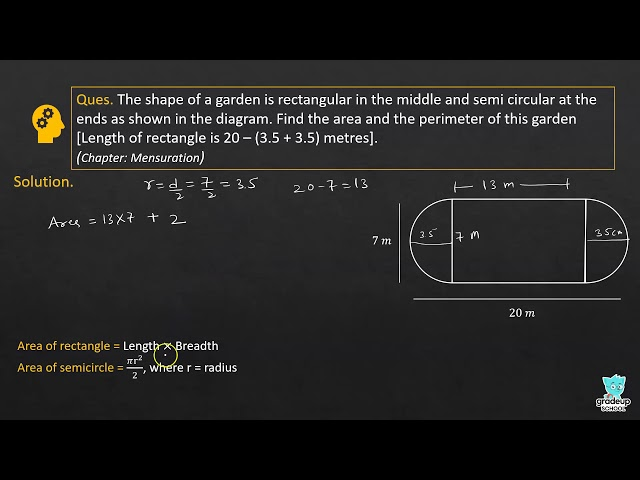# NCERT Solutions for Class 8 Maths Chapter 11 - Mensuration

Share

NCERT Mathematics Solutions for Class 8 Chapter 11- Mensuration prepared by subject experts in such a manner that students can easily understand the concepts of the topics and can solve the questions provided at the end of each Chapter. Designed by experts of Goprep, these NCERT Solutions are drafted in accordance with the latest official CBSE syllabus and in a well-structured and chapter-wise format for better understanding of the topics.

This Chapter deals with the important topics like Introduction to Mensuration, Previous lessons, Area of Trapezium, Area of a General Quadrilateral, Area of Special Quadrilateral, Area of Polygon, Solid Shapes, Surface Area of Cube, Surface Area of Cuboid and Cylinder, Volume and Capacity, Volume of Cube,  Volume of Cylinder and Cuboid etc. Apart from this, with the help of these Solutions, you will be able to form a relationship between the different geometrical shapes.

## NCERT Solutions for Class 8 Maths Chapter 11 - Mensuration

Exercises 11.1
• Exercises 11.1
• Exercises 11.2
• Exercises 11.3
• Exercises 11.4
Class 8th|NCERT - MathematicsChapter 11 - MensurationNCERT - MathematicsClass 8th , NCERT
 Chapter 1 - Rational Numbers Chapter 2 - Linear Equations in One Variable Chapter 3 - Understanding Quadrilaterals Chapter 4 - Practical Geometry Chapter 5 - Data Handling Chapter 6 - Squares and Square Roots Chapter 7 - Cubes and Cube Roots Chapter 8 - Comparing Quantities Chapter 9 - Algebraic Expressions and Identities Chapter 10 - Visualising Solid Shapes Chapter 11 - Mensuration Chapter 12 - Exponents and Powers Chapter 13 - Direct and Inverse Proportions Chapter 14 - Factorisation Chapter 15 - Introduction to Graphs Chapter 16 - Playing with Numbers